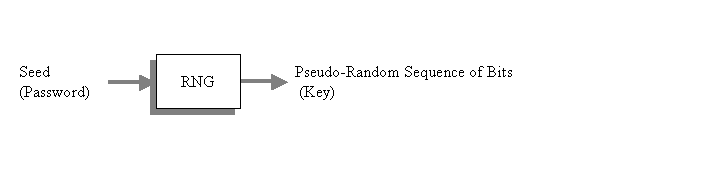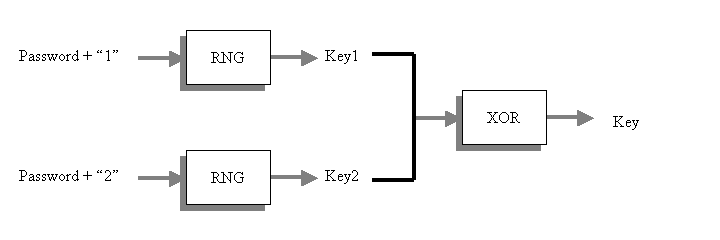# Key derivation using random number generators

Martin McBride, 2017-04-09
Tags cryptography key derivation random numbers
Categories cryptography

A psuedo-random number generator (RNG) is an algorithm which generates a stream of bits which have highly random statistical properties. The algorithm accepts a seed, in the form of an arbitrary length byte string. Any particular seed will always generate the same bit sequence, but different seeds will generate different sequences.

The application of RNG to key derivation is quite simple. The password is used as the seed value, and the bit sequence produced by the algorithm is used as the key. The advantage of this technique over hashing is that the key can be arbitrarily large (you can just keep on reading bits from the sequence).## The search space problem

The internal operation of many RNG algorithms acts to seriously restrict the search space.

What happens in some algorithms is that the initial seed is effectively hashed to quite a short length, eg 64 bits, before it is used to create the random sequence. This limitation usually arises, unavoidably, out of the structure of the algorithm.

What this means is that the RNG will accept a very long password, and produce a very long key, but the small search space means that you will not be getting the level of security you are expecting. For example, you can create a 1024 bit key, but the algorithm will only produce 264 different 1024 bit keys, whatever password you supply.

## Solving the problem

A solution to this problem is specified in PKCS#5. We describe a simplified version here.Here is the scheme. We take the original password, append the string "1" to the end of it, and use that string to seed the RNG. We use this to generate Key1, of the required bitlength (eg 1024 bits).

Then, we take the original password, append the string "2" to the end of it, and generate Key2 in a similar way.

Finally, we XOR the 2 values together to create the final key - this key has a much larger search space.

How does this work? We know that Key1 must belong to the 264 possible keys which make up the RNG search space. We also know that Key2 belongs to the same search space. However, Key1 and Key2 are both, in effect, random bit streams, then when you XOR the two together you will get a thirs random bit stream which almost certainly does not belong to the same search space.

If you were to analyse a very, very large collection of passwords (much larger than the search space of 264), you would eventually find 2 passwords, PasswordA and PasswordB, such that PasswordA had the same Key1 as PasswordB (ie the RNG of PasswordA + "1" is the same as the RNG of PasswordB + "1"). However, due to the pseudo-random nature of RNG algorithms, it is almost certain that the Key2 value would be different for the 2 passwords. In fact, if you analysed a vast number of passwords, you would almost every combination of possible values for Key1 and Key2, and almost every one of these combinations would create a unique key.

If you consider that Key1 has 264 possible values, and Key2 also has 264 possible values, then the total number of combinations of the XORed key is 2128.

Of course, this method can be readily extended to create a Key3, Key4, Key5 etc, which are all XORed together to create the final key. Each extra stage adds about 64 bits to the search space, so after 16 stages or more the search space would be adequate to generate truly secure 1024 bit keys.

## Salting and Iterations

[[data formats:cryptography:Salting|Salting]] is advisable for this algorithm, just as it is for the hash based key derivation. Simply combine the salt with the password before executing the algorithm.

In the case of the hash based, we described the technique of using many iterations of the algorithm to increase its execution time (to make it more difficult for a hacker to mount a dictionary attack). In this case, a better technique is to increase the number of keys calculated. There is no need to limit the calculation to just a few keys. You can use thousands of keys without any detriment to security.

Copyright (c) Axlesoft Ltd 2021